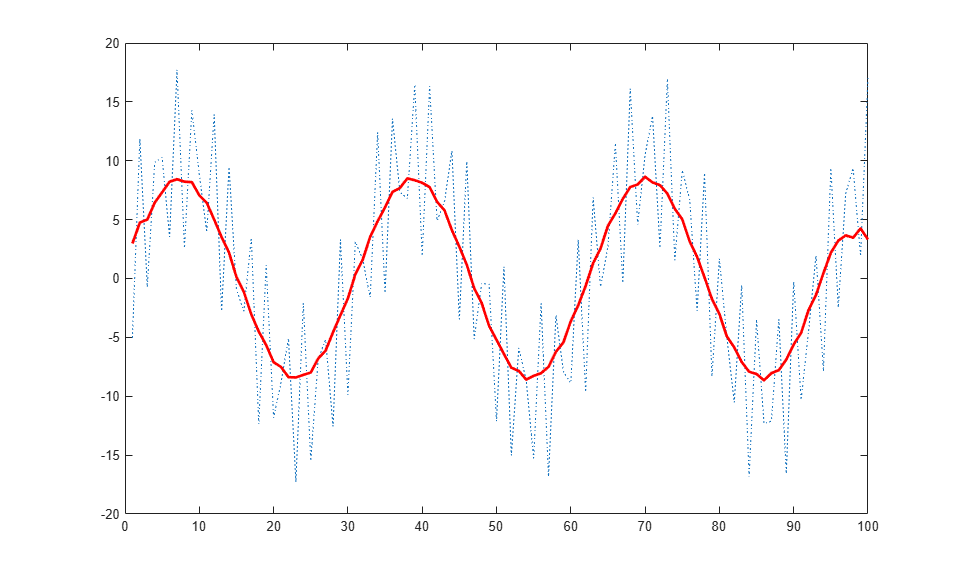setup

Class: matlab.graphics.chartcontainer.ChartContainer
Package: matlab.graphics.chartcontainer

Set up instance of chart container subclass

setup(obj)

Description

setup(obj) sets the initial state of the chart. It executes once when the chart object is created. Any property values passed as name-value pair arguments to the chart's constructor method are assigned after the setup method executes.

Define this method to execute initialization code for each new instance of your class. For example, you can use this method to create the underlying graphics objects and set initial property values on those objects.

Input Arguments

expand all

Object of the class that inherits from the matlab.graphics.chartcontainer.ChartContainer base class.

Attributes

 Abstract true Protected true

To learn about attributes of methods, see Method Attributes.

Examples

expand all

Define a class called SmoothPlot that plots a set of data using a dotted blue line with a smoothed version of the line.

To define the class, create a file called SmoothPlot.m that contains the following class definition with these features:

• XData and YData public properties that store the x- and y-coordinate data for the original line.

• SmoothColor and SmoothWidth public properties that control the color and width of the smooth line.

• OriginalLine and SmoothLine private properties that store the Line objects for original and smoothed data.

• A setup method that initializes OriginalLine and SmoothLine.

• An update method that updates the plot when the user changes the value of a property.

• A createSmoothData method that calculates a smoothed version of YData.

classdef SmoothPlot < matlab.graphics.chartcontainer.ChartContainer
properties
XData (1,:) double = NaN
YData (1,:) double = NaN
SmoothColor (1,3) double {mustBeGreaterThanOrEqual(SmoothColor,0),...
mustBeLessThanOrEqual(SmoothColor,1)} = [0.9290 0.6940 0.1250]
SmoothWidth (1,1) double = 2
end
properties(Access = private,Transient,NonCopyable)
OriginalLine (1,1) matlab.graphics.chart.primitive.Line
SmoothLine (1,1) matlab.graphics.chart.primitive.Line
end

methods(Access = protected)
function setup(obj)
% Create the axes
ax = getAxes(obj);

% Create the original and smooth lines
obj.OriginalLine = plot(ax,NaN,NaN,'LineStyle',':');
hold(ax,'on')
obj.SmoothLine = plot(ax,NaN,NaN);
hold(ax,'off')
end
function update(obj)
% Update line data
obj.OriginalLine.XData = obj.XData;
obj.OriginalLine.YData = obj.YData;
obj.SmoothLine.XData = obj.XData;
obj.SmoothLine.YData = createSmoothData(obj);

% Update line color and width
obj.SmoothLine.Color = obj.SmoothColor;
obj.SmoothLine.LineWidth = obj.SmoothWidth;
end
function sm = createSmoothData(obj)
% Calculate smoothed data
v = ones(1,10)*0.1;
sm = conv(obj.YData,v,'same');
end
end
end

Next, create a pair of x and y vectors. Plot x and y by calling the SmoothPlot constructor method, which is provided by the ChartContainer class. Specify the 'XData' and 'YData' name-value pair arguments and return the object as c.

x = 1:1:100;
y = 10*sin(x./5) + 8*sin(10.*x + 0.5);
c = SmoothPlot('XData',x,'YData',y);Use c to change the color of the smooth line to red.

c.SmoothColor = [1 0 0];Compatibility Considerations

expand all

Behavior changed in R2020a

Introduced in R2019b